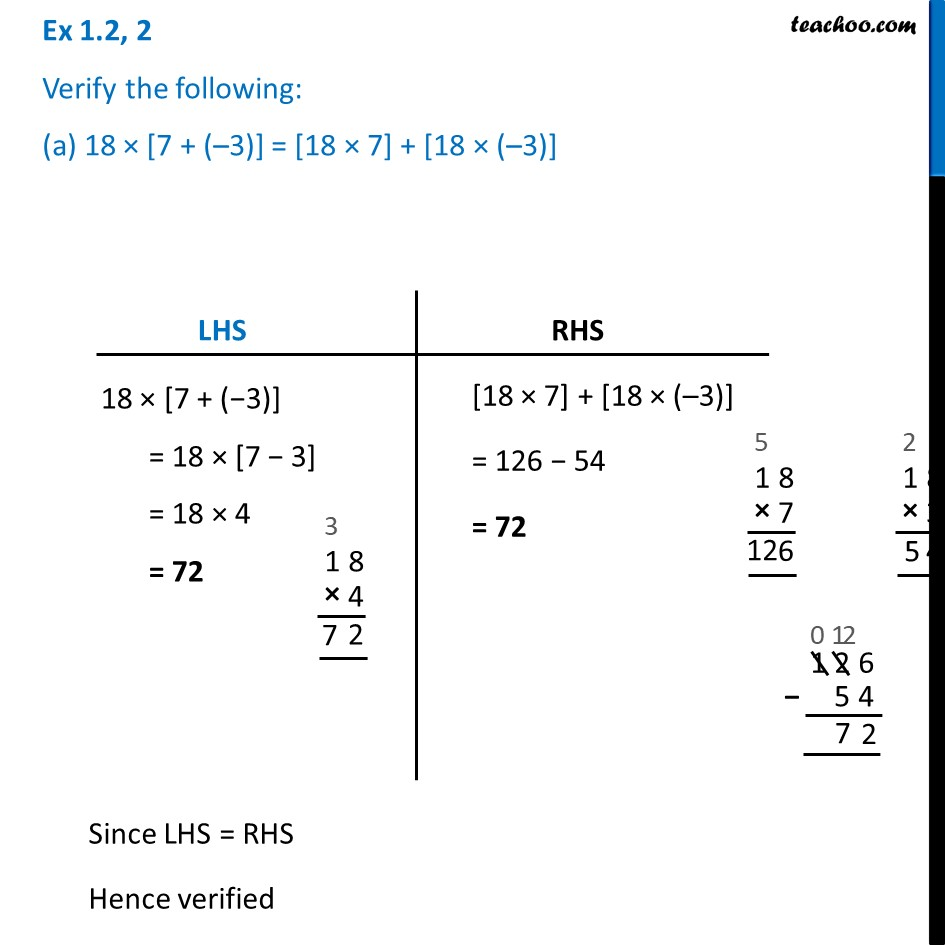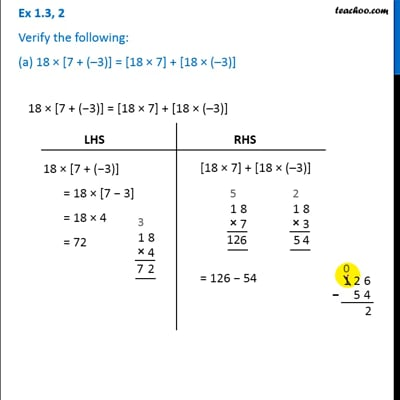Ex 1.2

Chapter 1 Class 7 Integers
Serial order wiseThis video is only available for Teachoo black users

Learn in your speed, with individual attention - Teachoo Maths 1-on-1 Class

### Transcript

Ex 1.2, 2 Verify the following: (a) 18 × [7 + (–3)] = [18 × 7] + [18 × (–3)] 18 × [7 + (−3)] = 18 × [7 − 3] = 18 × 4 = 72 18 × [7 + (−3)] = 18 × [7 − 3] = 18 × 4 = 72 Since LHS = RHS Hence verified